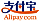Borítókép a Overdetermined system - hoz
Könyv címe:

# Overdetermined system

Betascript Publishing (06.06.2010 )Érvényes utalvány
ISBN-13:

### 978-613-0-33916-6

ISBN-10:
613033916X
EAN:
9786130339166
Könyv nyelve:
angol
Ismertető:
Please note that the content of this book primarily consists of articles available from Wikipedia or other free sources online. In mathematics, a system of linear equations is considered overdetermined if there are more equations than unknowns. The terminology can be described in terms of the concept of counting constants. Each unknown can be seen as an available degree of freedom. Each equation introduced into the system can be viewed as a constraint that restricts one degree of freedom. Therefore the critical case occurs when the number of equations and the number of independent variables are equal. For every degree of freedom, there exists a corresponding restraint. The overdetermined case occurs when the system has been overconstrained—that is, when the number of equations outnumbers the number of the unknowns.
Betascript Publishing
Weboldal:
https://www.betascript-publishing.com/
Szerkesztette:
Lambert M. Surhone, Miriam T. Timpledon, Susan F. Marseken
Oldalszám:
88
06.06.2010
Raktárkészlet:
Raktáron
Kategória:
Matematika
Ár:
34,00 €
Kulcsszavak:
Mathematics, System of Linear Equations, Codimension, variable, Degrees of Freedom, Cartesian Coordinate System, Consistency, Linear Independence, Row Space, Column Space### Kategóriák